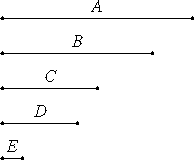# Proposition 22

The least numbers of those which have the same ratio with them are relatively prime.

Let A and B be the least numbers of those which have the same ratio with them.

I say that A and B are relatively prime.

If they are not relatively prime, then some number C measures them.

Let there be as many units in D as the times that C measures A, and as many units in E as the times that C measures B.Let there be as many units in D as the times that C measures A, and as many units in E as the times that C measures B.

VII.Def.15

Since C measures A according to the units in D, therefore C multiplied by D makes A. For the same reason C multiplied by E makes B.

VII.17

Thus the number C multiplied by the two numbers D and E makes A and B, therefore D is to E as A is to B.

Therefore D and E are in the same ratio with A and B, being less than they, which is impossible. Therefore no number measures the numbers A and B.

Therefore A and B are relatively prime.

Therefore, the least numbers of those which have the same ratio with them are relatively prime.

Q.E.D.

## Guide

This proposition is the converse of the last one. Together they say that a ratio a : b is reduced to lowest terms if and only if a is relatively prime to b.

#### Use of this proposition

This proposition is used in propositions VIII.2, VIII.3, and IX.15.